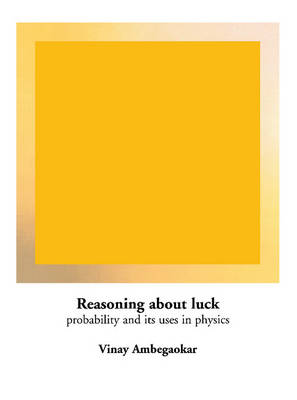•# Reasoning about Luck: Probability and its Uses in Physics (Paperback)

(author)
£34.99
Paperback 248 Pages / Published: 13/07/1996
• Not available

This product is currently unavailable

This product is currently unavailable.

This book introduces the reader to statistical reasoning and its use in physics. It is based on a course taught to non-science majors at Cornell, and differs from other treatments by its wide-ranging use of quantitative methods, which are built up in a constructive way and assume only that the reader can add, subtract, multiply and divide with confidence. The author begins with a self-contained introduction to the everyday uses of probability, including the quantitative assessment of statistical information. Following a chapter on useful mathematical concepts, he develops the basic ideas of mechanical motion, the molecular theory of gases, entropy as a measure of molecular agitation, limitations on the conversion of heat to work, the physics of the direction of time, chaos, and the role of probability in quantum mechanics. To aid self-instruction, there are solved problems at the end of each chapter.

Publisher: Cambridge University Press
ISBN: 9780521447379
Number of pages: 248
Weight: 512 g
Dimensions: 247 x 174 x 14 mm